June 28, 2022

# Remainder Theorem

Remainder theorem

# Zeros of polynomials:

The value of a polynomial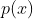at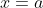is  obtained by puttinginand is denoted by  .

e.g.  Find the value of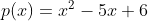at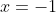.

Sol. On  puttingin given polynomial , we get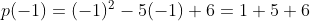This implies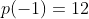.

Thus value ofatis 12.

We say that a zero of a polynomialis a number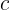such that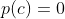.  It meansis  zero of  a polynomialif the value of that polynomial atis  zero.

The zeros of polynomials is obtained by  equating  the given polynomial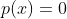.  We say  is a polynomial equation and is root of the polynomial equation .

Let  be a constant polynomial, then .

Now replace   with any number  we still get . This implies constant polynomials has no zeros.  In case of zero polynomial , every real number is a zero of the zero polynomial.

Important observations:

(i) Every linear polynomial has one and only one zero.

Let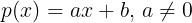be a linear polynomial,

thenmeans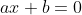.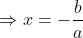So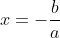is the only zero of.

i.e.  a linear  linear polynomial has one and only one zero.

(ii) A zero of polynomial need not be 0.

e. g.  The zeros of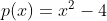are -2 and 2.

(iii) 0 may be a zero of polynomial.

e.g.  Take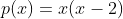(iv) A polynomial can have  more than one zero.

## Division algorithm in polynomials:

When we divide two numbers, we always get

Dividend =(divisor x quotient)+remainder,   where  .  When remainder becomes zero, we say divisor and quotient both are factors of dividend.

Now, let two polynomials   and . Divide  by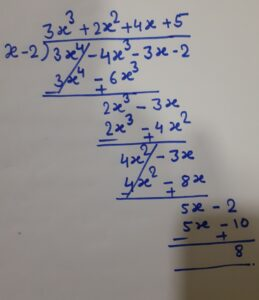{ Steps to divide a polynomial by a non-zero polynomial

• First, arrange the polynomials (dividend and divisor) in the decreasing order of its degree
• Divide the first term of the dividend by the first term of the divisor to produce the first term of the quotient
• Multiply the divisor by the first term of the quotient and subtract this product from the dividend, to get the remainder.
• This remainder is the dividend now and divisor will remain same
• Again repeat from the first step, until the degree of the new dividend is less than the degree of the divisor.}

Now

Hence    where  .

In general , If and are two polynomials  such that   and , then we can find a polynomial as quotient  and as remainder, where   or .

In the above example the divisior is a linear polynomial . In such a situation there is a way to find the  remainder called Remainder Theorem.

### Remainder theorem

Let be any polynomial of degree greater than or equal to one  and let be any real number. If  is divided by the  linear polynomial  , then remainder is  .

Proof. Let be any polynomial of degree greater than or equal to 1. Suppose is divided by then by using division algorithm theorem , can be written as

since degree of degree of ,  this implies degree of =0  ( degree of )

(a constant polynomial)

In particular if   then    which proves the theorem.

e.g. Find the remainder when is divided by .

Sol. Here and zeros of is 1.

so

=

Hence by the remainder theorem , the remainder is 2.